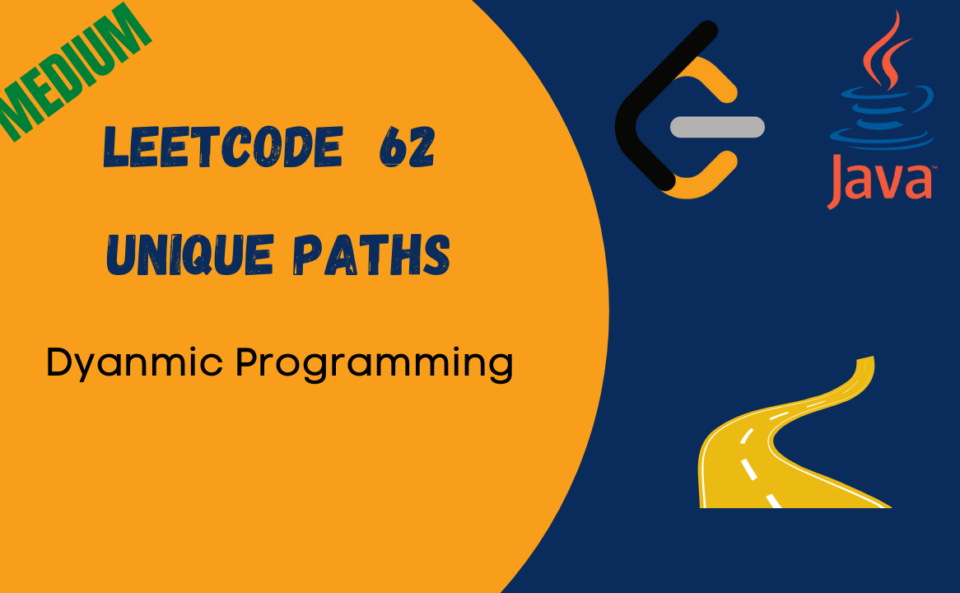# Leetcode Unique Paths Java SolutionA robot is located at the top-left corner of a m x n grid (marked ‘Start’ in the diagram below).

The robot can only move either down or right at any point in time. The robot is trying to reach the bottom-right corner of the grid (marked ‘Finish’ in the diagram below).

How many possible unique paths are there?

Example 1:

```Input: m = 3, n = 2
Output: 3
Explanation:
From the top-left corner, there are a total of 3 ways to reach the bottom-right corner:
1. Right -> Right -> Down
2. Right -> Down -> Right
3. Down -> Right -> Right
```

Example 2:

```Input: m = 7, n = 3
Output: 28```

Solution:
We will solve the problem using Dynamic Programming, adding the unique paths the pervious index to the left and previous index to the top and return the value in the final index.

``````class HackerHeap {
public int uniquePaths(int m, int n) {
int[][] dp = new int[m][n];

for(int i=0;i<m;i++){
for(int j=0;j<n;j++){
if(i==0||j==0) {
dp[i][j] =1;
}else {
dp[i][j] += dp[i-1][j] + dp[i][j-1];
}
}
}
return dp[m-1][n-1];
}
}``````